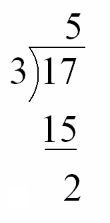# 揭開餘式定理 Remainder Theorem 的真面目

• 31/10/2010$$f(x) \equiv (ax+b)Q(x) + R$$

\begin{align*} f\Big(-\frac{b}{a}\Big) &= \Big(a(-\frac{b}{a}) + b\Big)Q\Big(-\frac{b}{a}\Big) + R\\ f\Big(-\frac{b}{a}\Big) &= (-b+b)Q\Big(-\frac{b}{a}\Big) + R\\ f\Big(-\frac{b}{a}\Big) &= R\\ R &= f\Big(-\frac{b}{a}\Big) \end{align*}

∴ 餘式 remainder = ##f\big(-\frac{b}{a}\big)##

「被除數 ≡ 除數 × 商 + 餘數」這最基本的恆等式才能找到答案。

Let f(x) be a polynomial. If f(x) is divisible by x + 2, which of the following must be a factor of f(3x + 1)?
f(x) 為一多頂式。若 f(x) 可被 x + 2 整除，則下列何者必為 f(3x + 1) 的因式?

A. x + 1
B. x + 2
C. 3x + 1
D. 3x – 1

Let the quotient be Q(x) when f(x) is divided by x + 2.
f(x) 除以 x + 2 時的商為 Q(x)

\begin{align*} f(x) &= (x\ +\ 2)\cdot Q(x)\\ \\ f(3x + 1) &= (3x + 1\ + 2)\cdot Q(3x+1)\\ f(3x + 1) &= (3x+3)\cdot Q(3x+1)\\ f(3x + 1) &= 3(x+1)\cdot Q(3x+1)\\ \end{align*}

HKCEE 2007 Paper II Q40 和以上例題非常相似，而該題答對百分比只有 19%，所以大家必須加倍留意！

「被除數 ≡ 除數 × 商 + 餘數」 才是餘式定理的真面目

It is given that f(x) = 2x3 – 5x2 + 4x – 8. Find the remainder when f(x + 3) is divided by x2 + 2x – 3.

### 回應 (4)

1.Edward 說：

點解餘式要設做mx + n?

•Thomas Fok 說：

餘式的次數(Degree)必定小於被除式的次數。被除式是二次函數，所以其餘式的次數最多為 1，即是 x 最多只為 1 次方，例子是 2x-3, 3x, 5 或 0。所以餘式必定能夠以 mx+n 形式表示。

•小白 說：

但dividend唔係x+3咩，一次黎wor
咁係睇dividend or 睇divider degree

•Thomas Fok 說：

Dividend is f(x+3), not x+3.

$$\because f(x)=2x^3 – 5x^2 + 4x – 8\\ \therefore f(x+3)\text{ is cubic function.}$$